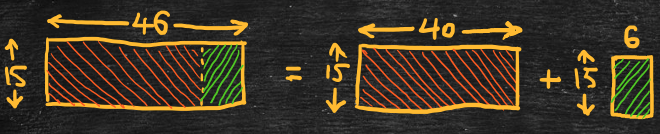Part 3: Multiplication

# 3.2: Expressions involving multiplication and addition

Notes

##### Applying the distributive property

To make a multiplication problem easier to solve, we can apply the distributive property to create an expression involving both multiplication and addition. For example,

• 46 x 15
• = (40 + 6) x 15
• = (40 x 15) + (6 x 15)
• = 600 + 90
• = 690

We can illustrate this by using the area model for multiplication. To calculate the area of a 46 by 15 rectangle, we can add the areas of a 40 by 15 rectangle and a 6 by 15 rectangle.For an example using an expression involving both multiplication and subtraction,

• 38 x 15
• = (40 – 2) x 15
• = (40 x 15) – (2 x 15)
• = 600 – 30
• = 570

For an example where we expand the integer being multiplied,

• 50 x 46
• = 50 x (40 + 6)
• = (50 x 40) + (50 x 6)
• = 2000 + 300
• = 2300

For an example that uses the distributive property in reverse,

• 27 + 18 + 36
• = (9 x 3) + (9 x 2) + (9 x 4)
• = 9 x (3 + 2 + 4)
• = 9 x 9
• = 81

The video below works through some examples of applying the distributive property in expressions involving multiplication and addition/subtraction.

Practice Exercises

Do the following exercises to practice applying the distributive property in expressions involving addition/subtraction.